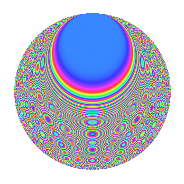# Properties

 Label 2016.2.dhLevel 2016 Weight 2 Character orbit dh Rep. character $$\chi_{2016}(943,\cdot)$$ Character field $$\Q(\zeta_{6})$$ Dimension 184 Sturm bound 768

# Related objects

## Defining parameters

 Level: $$N$$ = $$2016 = 2^{5} \cdot 3^{2} \cdot 7$$ Weight: $$k$$ = $$2$$ Character orbit: $$[\chi]$$ = 2016.dh (of order $$6$$ and degree $$2$$) Character conductor: $$\operatorname{cond}(\chi)$$ = $$504$$ Character field: $$\Q(\zeta_{6})$$ Sturm bound: $$768$$

## Dimensions

The following table gives the dimensions of various subspaces of $$M_{2}(2016, [\chi])$$.

Total New Old
Modular forms 800 200 600
Cusp forms 736 184 552
Eisenstein series 64 16 48

## Trace form

 $$184q + 6q^{3} - 2q^{9} + O(q^{10})$$ $$184q + 6q^{3} - 2q^{9} + 4q^{11} - 12q^{17} + 12q^{19} + 148q^{25} - 6q^{33} + 18q^{35} + 4q^{43} - 2q^{49} + 54q^{51} - 20q^{57} + 6q^{59} - 18q^{65} - 2q^{67} - 12q^{73} + 36q^{75} - 10q^{81} + 60q^{83} - 36q^{89} - 20q^{91} + 26q^{99} + O(q^{100})$$

## Decomposition of $$S_{2}^{\mathrm{new}}(2016, [\chi])$$ into newform subspaces

The newforms in this space have not yet been added to the LMFDB.

## Decomposition of $$S_{2}^{\mathrm{old}}(2016, [\chi])$$ into lower level spaces

$$S_{2}^{\mathrm{old}}(2016, [\chi]) \cong$$ $$S_{2}^{\mathrm{new}}(504, [\chi])$$$$^{\oplus 3}$$

## Hecke Characteristic Polynomials

There are no characteristic polynomials of Hecke operators in the database